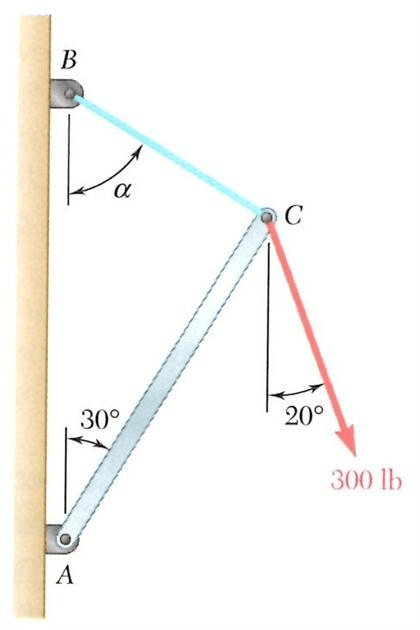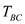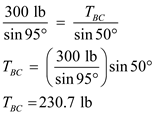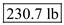# Knowing that α = 55° and that boom AC exerts on a pin C a force directed along line AC, determine a) the magnitude of that force b) The tension in cable BC.

Question-AnswerCategory: Engineering MechanicsKnowing that α = 55° and that boom AC exerts on a pin C a force directed along line AC, determine a) the magnitude of that force b) The tension in cable BC.

Knowing that α = 55° and that boom AC exerts on a pin C a force directed along line AC, determine
a) the magnitude of that force
b) The tension in cable BC.Step: 1

Draw free body diagram at point C as shown:Step: 2

From the Lami’s theorem, write the equilibrium equation of forces as follows:…… (1)
Here,is the tension in the cable BC andis the force in the member AC.

Step: 3

(a)
Calculate the force in the member AC as follows:
From equation (1),Here, the negative sign indicates that the original direction is opposite to that of assumed direction.
Therefore, the force in the member AC isStep: 4

(b)
Calculate the tension in the cable BC as follows:
From equation (1),Therefore, the tension in the cable BC is.

If you like the solution share it with your friends. & Subscribe Mazurek Gravity on YouTube.